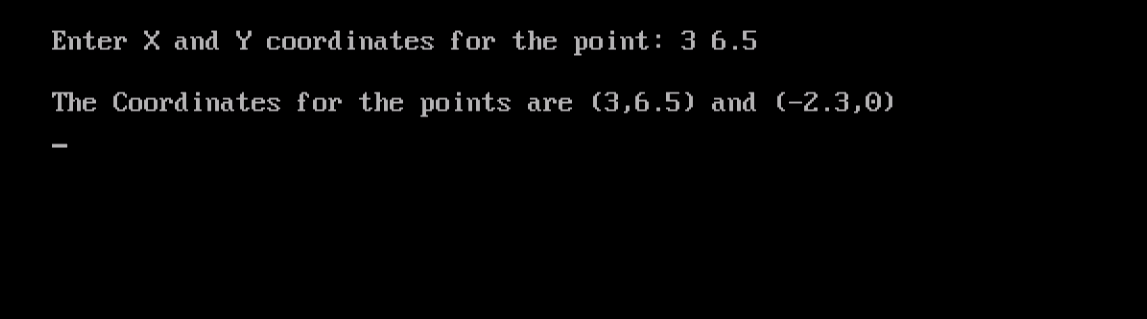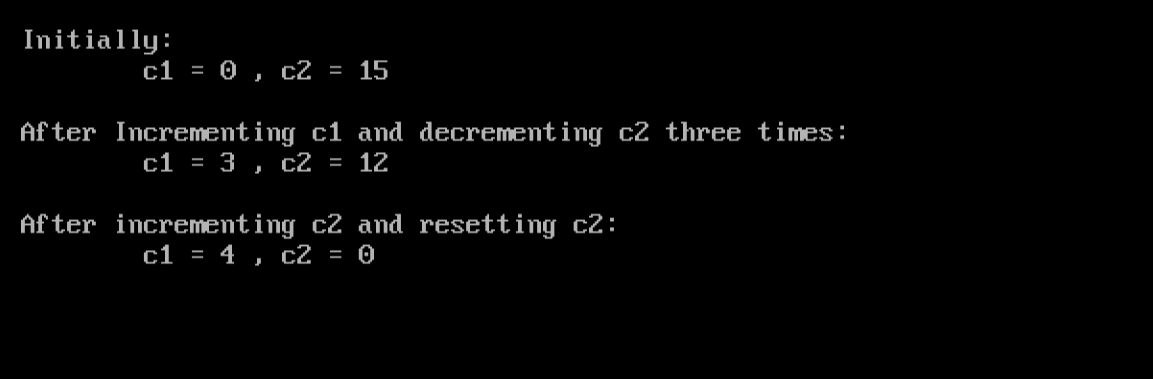# Learn about Constructors as Object Oriented Programming in C++

0
2294After introducing the Object-Oriented Programming concept: what it is, and what it is used for. And after we wrote our first Class program, we are going to build up on what we have learned in the previous article and go an extra mile. So, bear with us, and you won’t regret it.

Always Remember
A Class can be thought of like a model, pattern, or blueprint that specifies the attributes (variables) and methods (functions) that will exist in the instances (objects) of that class.
An Object is an instance of a defined class. It is the actual implementation of the sketched blueprint.

### Constructors

As mentioned in the previous article that the relation between an object and a class is analogous to that between a variable and the data type it belongs to. As you initialize a variable by assigning an initial value to it, there should be also a way to initialize an object. For this reason, Constructors comes to the scene.

I could use the set methods to assign values to the member variables, so why complicate things by using  “Constructors”?!

Actually, Constructors were introduced to simplify things, not to complicate it as you may think. Refer to the example in the previous article. We have created the P object of the class point.

`point P;`

Then, we used the setx() and sety() member functions to set values for the member variables x and y:

```P.setx(x1);
P.sety(y1);
```

So, three statements were required. That is what we used in our first class program, and this is exactly the way you have just suggested to initialize the data items in an object.

– Yes, that is exactly what I mean.

Okay, it is a good way. However, don’t you agree with me that it would be much better if we could do the object creation and initialization steps in one single statement instead of three?

– Mmm…Yes, it would be better, but how?!

Constructors!!!

### What are Constructors?

A Constructor is a public member function, whose name is the same as the class name, which is called automatically on object creation.

Example
In this example, we are going to re-write the point class to allow the initialization of objects when created.

Consider the following modified version:

```#include <iostream.h>
#include <conio.h>
class point
{
float x,y;
public:
point (float i, float j)
{
x=i;
y=j;
}
void setx(float i)
{ x=i; }
void sety(float j)
{ y=j; }
float getx()
{ return x; }
float gety()
{ return y; }
};
void main()
{
clrscr();
float x1,y1;
cout << "\nEnter X and Y coordinates for the point: ";
cin >> x1 >> y1;
point P1(x1,y1);
point P2(-2.3,0);
cout << "\nThe Coordinates for the points are (" << P1.getx()
<< "," << P1.gety() << ")" << " and (" << P2.getx()
<< "," << P2.gety() << ")" << endl;
getch();
}
```

When executed, the program should behave as follows:The class definition has been modified by adding the constructor function definition:

```point (float i, float j)
{
x=i;
y=j;
}
```

The constructor is a member function that is given the same name as the class in which it is defined (point in this case). The following rules must be taken into account when defining a constructor for a class:

• The constructor must be public.
• The constructor can’t specify a return type, even void.

The other modification is in the main() function:

```point P1(x1,y1);
point P2(-2.3,0);
```

Objects P1 and P2 are created and initialized in one step (for each).As a function, a constructor can be overloaded. This is useful to provide more than on way to initialize an object.

Example
In this example, we are going to write a program that implements the counter functionality. The counter could be initialized to any positive integer value. If not initialized, the counter is set to zero. The counter should have a set of member functions that increment, decrement, reset (set to zero), and retrieve its value.

Consider the following code:

```#include <conio.h>
#include <iostream.h>
class counter
{
private:
int c;
public:
counter(int val)
{ c = val; }
counter()
{ c = 0; }
int getCount()
{ return c; }
void increment()
{ c++; }
void decrement()
{ c--; }
void reset()
{ c = 0; }
};
void main()
{
clrscr();
counter c1;
counter c2(15);
cout << "\nInitially:\n\tc1 = " << c1.getCount() << " , c2 = "
<< c2.getCount() << endl;
for(int i=0;i<3;i++)
{ c1.increment(); c2.decrement(); }
cout << "\nAfter Incrementing c1 and decrementing c2 three times:\n"
<< "\tc1 = " << c1.getCount() << " , c2 = " << c2.getCount()
<< endl;
c1.increment(); c2.reset();
cout << "\nAfter incrementing c2 and resetting c2:\n"
<< "\tc1 = " << c1.getCount() << " , c2 = " << c2.getCount()
<< endl;
getch();
}
```

Let’s see it in action:In this program, two constructors have been defined:

The one that expects an argument (initial value):

```counter(int val)
{ c = val; }
```

And the one that doesn’t have an argument:

```counter()
{ c = 0; }
```

Obviously, the decision to invoke the former or the latter depends on the object being created. For example, when the object c1 was created with no initial value provided:

`counter c1;`

the second constructor is called. One the other hand, when the object c2 was created with initial value:

`counter c2(15);`

the first constructor is automatically called.

Summary